# Centroid

In Geometry, the centroid is an important concept related to a triangle. A triangle is a three-sided bounded figure with three interior angles. Based on the sides and angles, a triangle can be classified into different types such as

• Scalene triangle
• Isosceles triangle
• Equilateral triangle
• Acute-angled triangle
• Obtuse-angled triangle
• Right-angled triangle

The centroid is an important property of a triangle. Let us discuss the definition of centroid, formula, properties and centroid for different geometric shapes in detail.

## Centroid Definition

The centroid is the centre point of the object. The point in which the three medians of the triangle intersect is known as the centroid of a triangle. It is also defined as the point of intersection of all the three medians. The median is a line that joins the midpoint of a side and the opposite vertex of the triangle. The centroid of the triangle separates the median in the ratio of 2: 1. It can be found by taking the average of x- coordinate points and y-coordinate points of all the vertices of the triangle.

### Centroid Theorem

The centroid theorem states that the centroid of the triangle is at 2/3 of the distance from the vertex to the mid-point of the sides.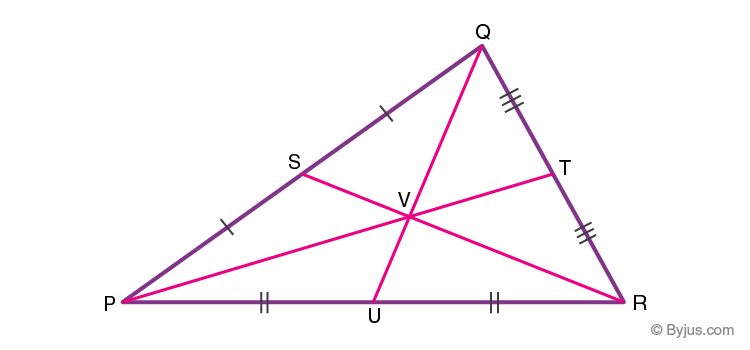Suppose PQR is a triangle having a centroid V. S, T and U are the midpoints of the sides of the triangle PQ, QR and PR, respectively. Hence as per the theorem;

QV = 2/3 QU, PV = 2/3 PT and RV = 2/3 RS

### Centroid of A Right Angle Triangle

The centroid of a right angle triangle is the point of intersection of three medians, drawn from the vertices of the triangle to the midpoint of the opposite sides.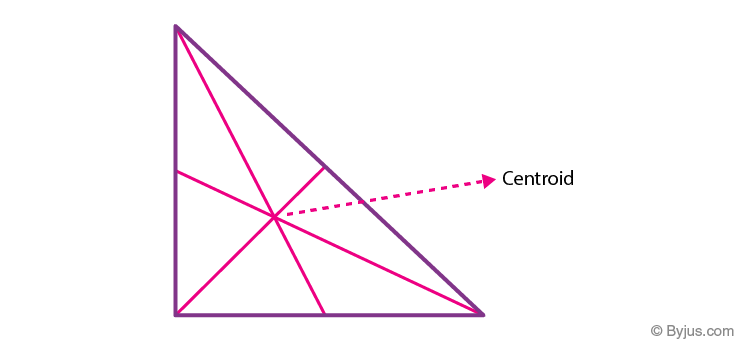### Centroid of a Square

The point where the diagonals of the square intersect each other is the centroid of the square. As we all know, the square has all its sides equal. Hence it is easy to locate the centroid in it. See the below figure, where O is the centroid of the square.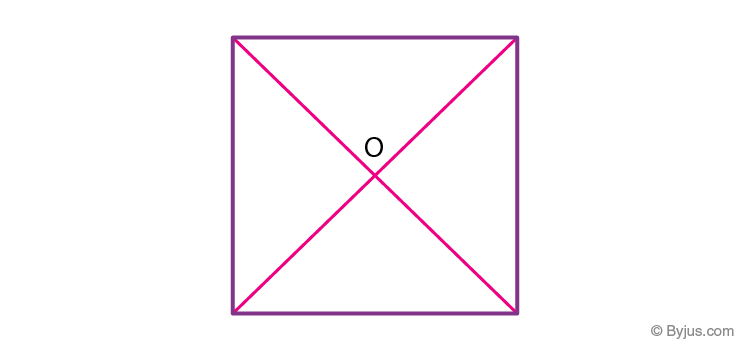## Properties of centroid

The properties of the centroid are as follows:

• The centroid is the centre of the object.
• It is the centre of gravity.
• It should always lie inside the object.
• It is the point of concurrency of the medians.

## Centroid Formula

Let’s consider a triangle. If the three vertices of the triangle are A(x1, y1), B(x2, y2), C(x3, y3), then the centroid of a triangle can be calculated by taking the average of X and Y coordinate points of all three vertices. Therefore, the centroid of a triangle can be written as:

Centroid of a triangle = ((x1+x2+x3)/3, (y1+y2+y3)/3)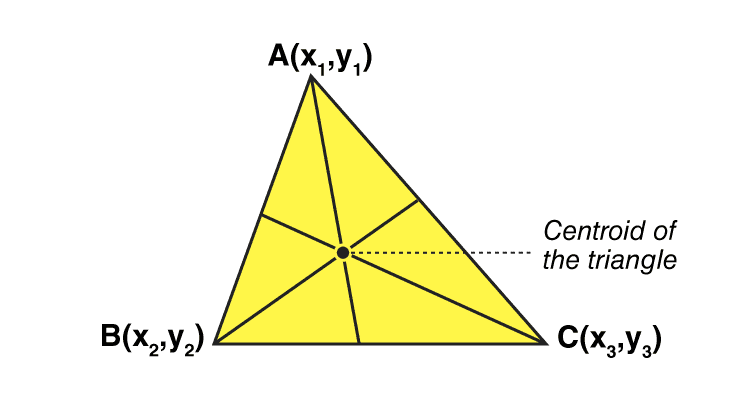### Centroid Formula For Different Shapes

Here, the list of centroid formula is given for different geometrical shapes.

 Shapes Figure x̄ ȳ Area Triangular area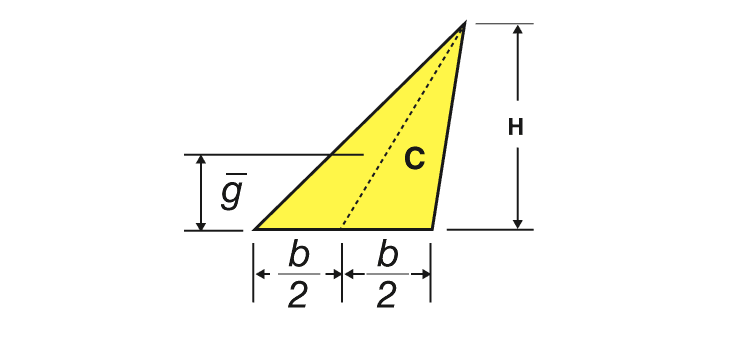– h/3 bh/2 Quarter-circular area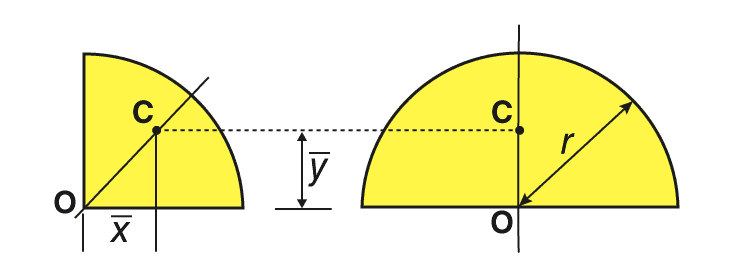4r/3π 4r/3π πr2/4 Semicircular area0 4r/3π πr2/2 Quarter-elliptical area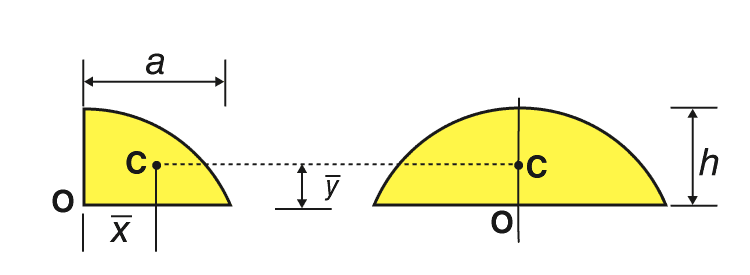4a/3π 4b/3π πab/4 Semi elliptical area0 4b/3π πab/2 Semiparabolic area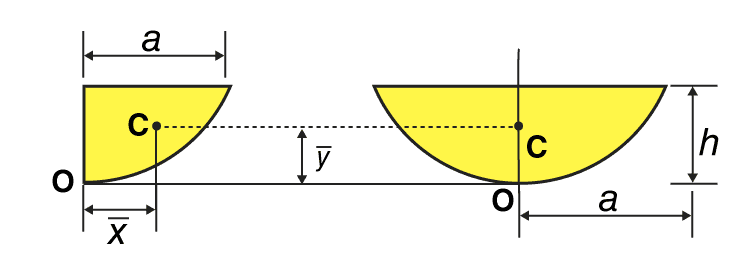3a/8 3h/5 2ah/3 Parabolic area0 3h/5 4ah/3 Parabolic spandrel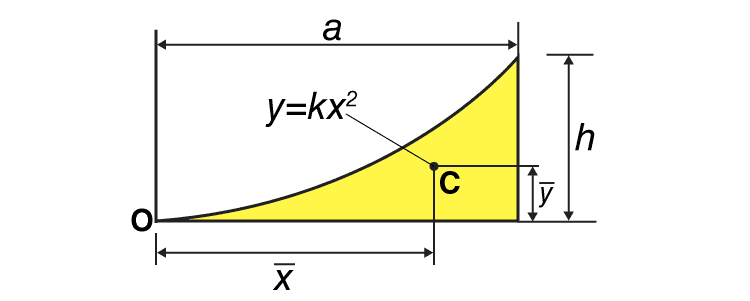3a/4 3h/10 ah/3

### Examples on Calculating Centroid

Find the solved examples below, to find the centroid of triangles with the given values of vertices.

Question 1: Find the centroid of the triangle whose vertices are A(2, 6), B(4, 9), and C(6,15).

Solution:

Given:

A(x1, y1) = A(2, 6)

B(x2, y2) = B(4,9)

C(x3, y3) = C(6,15)

We know that the formula to find the centroid of a triangle is = ((x1+x2+x3)/3, (y1+y2+y3)/3)

Now, substitute the given values in the formula

Centroid of a triangle = ((2+4+6)/3, (6+9+15)/3)

= (12/3, 30/3)

= (4, 10)

Therefore, the centroid of the triangle for the given vertices A(2, 6), B(4,9), and C(6,15) is (4, 10).

Question 2: Find the centroid of the triangle whose vertices are A(1, 5), B(2, 6), and C(4, 10).

Solution: Given, A(1, 5), B(2, 6), and C(4, 10) are the vertices of a triangle ABC.

By the formula of the centroid we know;

Centroid =  ((x1+x2+x3)/3, (y1+y2+y3)/3)

Putting the values, we get;

Centroid = (1+2+4)/3, (5+6+10)/3

=( 7/3, 21/3)

=( 7/3,7)

Hence, the centroid of the triangle having vertices A(1, 5), B(2, 6), and C(4, 10) is (7/3, 7).

Question 3: If the vertices of a triangle PQR is (2, 1), (3, 2) and (-2, 4). Then find the centroid of it.

Solution: Given, (2, 1), (3, 2) and (-2, 4) are the vertices of triangle pQR.

By the formula of the centroid we know;

Centroid =  ((x1+x2+x3)/3, (y1+y2+y3)/3)

Putting the values, we get;

Centroid, O = (2+3-2)/3, (1+2+4)/3

O = (3/3, 7/3)

O = (1, 7/3)

Hence, the centroid of the triangle having vertices (2, 1), (3, 2) and (-2, 4) is (1, 7/3).

Visit BYJU’S to learn different concepts on Maths and also download BYJU’S – The Learning App for personalised videos to learn with ease.